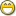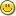### 1. How To Build Lookup Tables

In std/search.e, we have two functions for looking up items in a "table:" lookup() and vlookup().

Is anybody aware of any other functions for manipulating tables like this? I'm looking for functions like new, add, delete/remove, rename, etc...

Why have lookup and vlookup without the functionality to create the table in the first place? I know it's simple, but not when you have nested items hundreds of levels deep.

### 2. Re: How To Build Lookup Tables

euphoric said...

In std/search.e, we have two functions for looking up items in a "table:" lookup() and vlookup().

Is anybody aware of any other functions for manipulating tables like this? I'm looking for functions like new, add, delete/remove, rename, etc...

Why have lookup and vlookup without the functionality to create the table in the first place? I know it's simple, but not when you have nested items hundreds of levels deep.

Is the data actually sitting in nested sequences "hundreds of levels deep" or is it more like a 2-d table where there are hundreds of semantic levels?

Spock

### 3. Re: How To Build Lookup Tables

Spock said...

Is the data actually sitting in nested sequences "hundreds of levels deep" or is it more like a 2-d table where there are hundreds of semantic levels?

It's not actually that deep. But it could be, someday.

I wrote this for now (since I'm only using tables with two columns at the moment):

```namespace tables

-- functions for manipulating columnar data (tables)

public function new(sequence data = {{},{}})
return data
end function

public function lookup(object item, sequence data)
integer i = find(item,data)
if i = 0 then
return {0,{}}
end if
return {1,data[i]}
end function

public function set(sequence table, object key, object val = {})
integer i = find(key,table)
if i then
table[i] = val
else
table = append(table,key)
table = append(table,val)
end if
return table
end function

/* -- looks like <eucode> doesn't handle this comment block properly

include std/map.e
include std/console.e
sequence table = tables:new()

table = set(table,"a",123)
table = set(table,"b","Billy")
table = set(table,"c",{247,'x',{1,7,7}})
table = set(table,"x","Hawaii")
table = set(table,"y",77897)
table = set(table,"z",map:new())

display(lookup("c",table))

wait_key()
*/
```

Any enhancements are welcome!

### 4. Re: How To Build Lookup Tables

euphoric said...
```sequence table = tables:new()

table = set(table,"a",123)
table = set(table,"b","Billy")
table = set(table,"c",{247,'x',{1,7,7}})
table = set(table,"x","Hawaii")
table = set(table,"y",77897)
table = set(table,"z",map:new())
```

Congratulations, you've created really slow maps!What are you looking for here, exactly? I know one problem we discussed previously was that save_map doesn't know how to store sub-maps. Are you trying to get around that?

-Greg

### 5. Re: How To Build Lookup Tables

ghaberek said...
euphoric said...
```sequence table = tables:new()

table = set(table,"a",123)
table = set(table,"b","Billy")
table = set(table,"c",{247,'x',{1,7,7}})
table = set(table,"x","Hawaii")
table = set(table,"y",77897)
table = set(table,"z",map:new())
```

Congratulations, you've created really slow maps!I know!I'm just starting with the simple iteration.

ghaberek said...

What are you looking for here, exactly? I know one problem we discussed previously was that save_map doesn't know how to store sub-maps. Are you trying to get around that?

Yes. Ultimately, I'll have deeper-nested tables, and this will make it easier to load/save them without having to manually track what all is a map.

### 6. Re: How To Build Lookup Tables

euphoric said...

Yes. Ultimately, I'll have deeper-nested tables, and this will make it easier to load/save them without having to manually track what all is a map.

I should probably be using a Euphoria database...

### 7. Re: How To Build Lookup Tables

euphoric said...

I should probably be using a Euphoria database...

Would a relational database be appropriate?

-Greg

### 8. Re: How To Build Lookup Tables

ghaberek said...
euphoric said...

I should probably be using a Euphoria database...

Would a relational database be appropriate?

Yes, for sure.